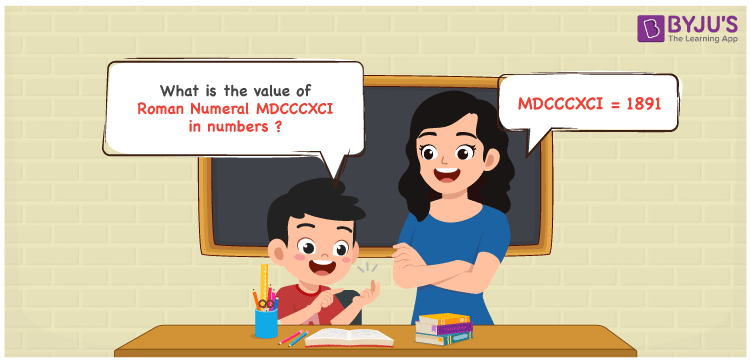Checkout JEE MAINS 2022 Question Paper Analysis : Checkout JEE MAINS 2022 Question Paper Analysis :

# MDCCCXCI Roman Numerals

MDCCCXCI Roman Numerals is 1891. This article aims to assist students with the conversion of MDCCCXCI to numbers. The explanation given in simple language improves the logical thinking skills among students irrespective of their grasping abilities. This Roman numerals article has all the concepts required to understand the conversion effectively. Therefore, MDCCCXCI can be indicated as 1891 in numbers.

 Number Roman Numeral 1891 MDCCCXCI## How to Write MDCCCXCV Roman Numerals in Numbers?

Learn how MDCCCXCI is transformed to numbers as 1891 using the steps below.

MDCCCXCI = M + D + C + C + C + (C – X) + I

MDCCCXCI = 1000 + 500 + 100 + 100 + 100 + (100 – 10) + 1

MDCCCXCI = 1891

## Video Lesson on Roman Numerals## Frequently Asked Questions on MDCCCXCI Roman Numerals

### Why is 1891 written as MDCCCXCI?

In roman numerals

1000 is written as M

800 is written as DCCC

90 is written as XC

1 is written as I

1891 = M + DCCC + XC + I = MDCCCXCI

### Find 1900 – 9.

We know that

1900 – 9 = 1891

Hence, 1900 – 9 is 1891 which is written as MDCCCXCI.

### Find the remainder we get when MDCCCXCI is divided by XVII.

We know that

MDCCCXCI = 1891

XVII = 17

So the remainder we get when MDCCCXCI is divided by XVII is IV.